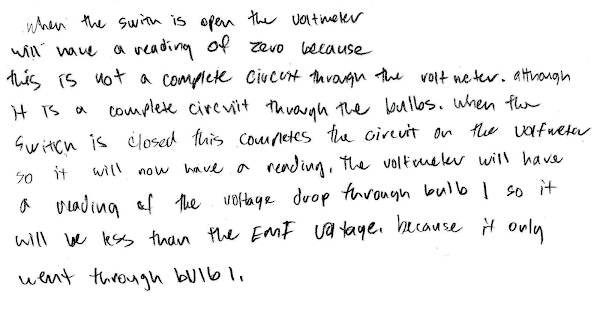## 20150624

### Physics final exam question: converging lens image same size as object

Physics 205B Final Exam, spring semester 2015
Cuesta College, San Luis Obispo, CA

Cf. Giambattista/Richardson/Richardson, Physics, 2/e, Problem 23.63

A Physics 205B student places a object 22 cm in front of (to the left of) a converging lens (f = +11 cm). Discuss why the size of resulting image will be exactly the same size as the original object. Explain your reasoning by using the properties of lenses, thin lens equations and/or ray tracings.

• p:
Correct. Image will be the same size as the original object because:
1. from the thin lens equation, the image distance di will be the same as the object image distance do;
2. and from the linear magnification equation, the image height h' will be –hdi/do, which makes the image the same size as (but inverted from) the original object.
• r:
As (p), but argument indirectly, weakly, or only by definition supports the statement to be proven, or has minor inconsistencies or loopholes. Typically shows that do = di, but does not explicitly relate this to how the image and object sizes compare.
• t:
Nearly correct, but argument has conceptual errors, or is incomplete.
• v:
Limited relevant discussion of supporting evidence of at least some merit, but in an inconsistent or unclear manner.
• x:
Implementation/application of ideas, but credit given for effort rather than merit.
• y:
Irrelevant discussion/effectively blank.
• z:
Blank.
Sections 30882, 30883
Exam code: finalLd0c
p: 16 students
r: 18 students
t: 2 students
v: 2 students
x: 1 student
y: 1 student
z: 3 student

A sample "p" response (from student 5425):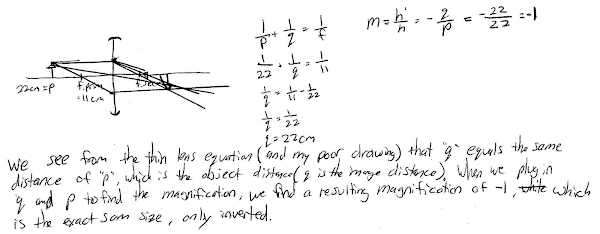A sample "r" response (from student 1675):### Physics final exam question: switching positions of ammeter and voltmeter

Physics 205B Final Exam, spring semester 2015
Cuesta College, San Luis Obispo, CA

Cf. Giambattista/Richardson/Richardson, Physics, 2/e, Problems 18.31, 18.41, 18.65A 1.50 V emf battery with an unknown internal resistance r is connected to a light bulb of resistance R, and a voltmeter and an ammeter. All of these components are ideal. The internal resistance r is less than the resistance R of the light bulb. Discuss in which circuit (a) or (b) will the ammeter have a greater reading, or if there will be a tie. Explain your reasoning using the properties of voltmeters and ammeters, Kirchhoff's rules and Ohm's law.

• p:
Correct. Understands that:
1. a voltmeter has an infinite resistance, such that in circuit (a) it allows the current to flow through both the battery (with its internal resistance r) and the light bulb (of resistance R), while in circuit (b) the voltmeter prevents current from flowing through the light bulb, and only through the battery itself;
2. from Ohm's law, the current flowing in circuit (a) is given by the emf of the battery divided by the sum of the resistances r and R, while in circuit (b) the current is given by the emf of the battery divided only the resistance r, such that the ammeter in (b) will have a higher reading than in the ammeter in (a).
• r:
As (p), but argument indirectly, weakly, or only by definition supports the statement to be proven, or has minor inconsistencies or loopholes. One of the two points (1)-(2) correct, other is problematic/incomplete.
• t:
Nearly correct, but argument has conceptual errors, or is incomplete. Only one of the two points (1)-(2) correct, other is missing, or both are problematic.
• v:
Limited relevant discussion of supporting evidence of at least some merit, but in an inconsistent or unclear manner.
• x:
Implementation/application of ideas, but credit given for effort rather than merit.
• y:
Irrelevant discussion/effectively blank.
• z:
Blank.
Sections 30882, 30883
Exam code: finalLd0c
p: 12 students
r: 5 students
t: 4 students
v: 12 students
x: 10 students
y: 2 students
z: 1 student

A sample "p" response (from student 5425):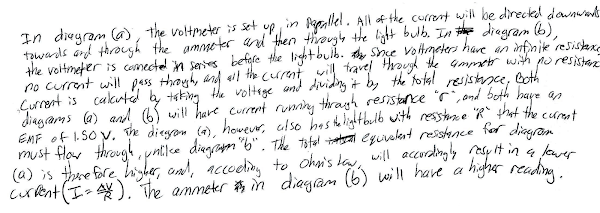A sample "v" response (from student 0621):### Physics final exam question: Cu-64 decay processes and daughter isotopes

Physics 205B Final Exam, spring semester 2015
Cuesta College, San Luis Obispo, CA

Cf. Giambattista/Richardson/Richardson, Physics, 2/e, Conceptual Question 29.4, Problem 29.26

Cu(64,29) ￼can either undergo either ￼β decay or electron capture. Discuss whether these two processes will both result in the same daughter isotope, or instead will result in two different types of daughter isotopes. Explain your reasoning using properties of nuclides and radioactive decay modes.

• p:
Correct. Compares the two decay processes:
1. β- decay emits an electron, in the process converting a neutron into a proton, which leaves the nucleon number the same, but increases the atomic number (resulting in zinc);
2. electron capture takes in an electron to covert a proton into a neutron, which also leaves the nucleon number the same, but decreases the atomic number (resulting in nickel); thus two different types of daughter elements (different proton numbers) result from these two decay processes. (The daughter elements need not be explicitly named, as long as the different proton numbers are recognized.)
• r:
As (p), but argument indirectly, weakly, or only by definition supports the statement to be proven, or has minor inconsistencies or loopholes.
• t:
Nearly correct, but argument has conceptual errors, or is incomplete.
• v:
Limited relevant discussion of supporting evidence of at least some merit, but in an inconsistent or unclear manner. Some garbled attempt at applying properties of radioactive decay.
• x:
Implementation/application of ideas, but credit given for effort rather than merit. Approach other than that of applying properties of radioactive decay.
• y:
Irrelevant discussion/effectively blank.
• z:
Blank.
Sections 30882, 30883
Exam code: finalLd0c
p: 16 students
r: 6 students
t: 5 students
v: 8 students
x: 2 students
y: 2 students
z: 4 students

A sample "p" response (from student 0104):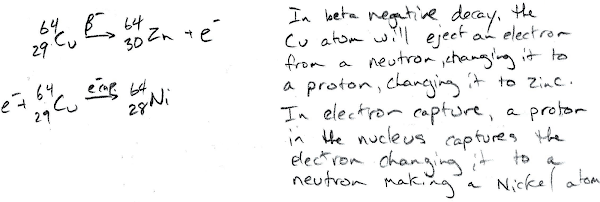### Physics final exam question: "resetting" solidification age of sample

Physics 205B Final Exam, spring semester 2015
Cuesta College, San Luis Obispo, CA

A rock sample with an extremely old solidification age (as determined by radioactive dating) is heated until it is molten, and then cooled back down to a solid. Discuss what happens to its solidification age (as determined by radioactive dating), and explain why this happens. Explain your reasoning using properties of radioactive decay.

• p:
Correct. Discusses:
1. the solidification age of a rock is determined by comparing the amount of gaseous decay products to the amount of unstable radioactive isotopes in the sample; a higher ratio of gaseous products to unstable isotopes corresponds to a sample that had solidified a long time ago; and
2. heating the rock until molten would release the gaseous decay products, such that when it cools back down to a solid, it would have nothing to compare to the unstable isotopes that remain in the sample, effectively giving it a zero solidification age.
• r:
As (p), but argument indirectly, weakly, or only by definition supports the statement to be proven, or has minor inconsistencies or loopholes. One of the two points (1)-(2) correct, other is problematic/incomplete.
• t:
Nearly correct, but argument has conceptual errors, or is incomplete. Only one of the two points (1)-(2) correct, other is missing, or both are problematic.
• v:
Limited relevant discussion of supporting evidence of at least some merit, but in an inconsistent or unclear manner. Some garbled attempt at applying properties of radioactive decay.
• x:
Implementation/application of ideas, but credit given for effort rather than merit. Approach other than that of applying properties of radioactive decay.
• y:
Irrelevant discussion/effectively blank.
• z:
Blank.
Sections 30882, 30883
Exam code: finalLd0c
p: 11 students
r: 13 students
t: 6 students
v: 10 students
x: 0 students
y: 1 student
z: 2 students

A sample "p" response (from student 3019):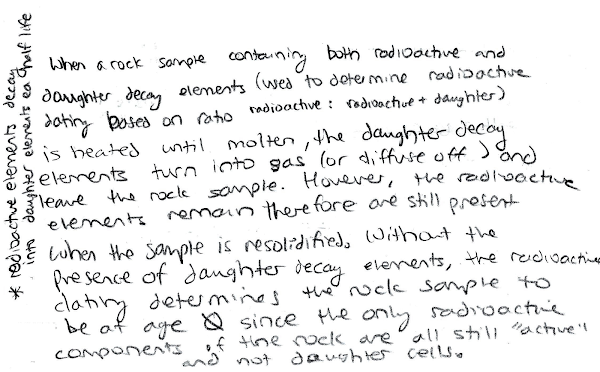Another sample "p" response (from student 5080):A sample "v" response (from student 1107):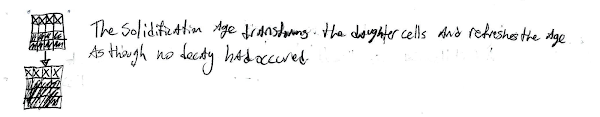### Physics final exam problem: interference of two in-phase Wi-Fi antennae

Physics 205B Final Exam, spring semester 2015
Cuesta College, San Luis Obispo, CA

Cf. Giambattista/Richardson/Richardson, Physics, 2/e, Problem 25.31"Wifi"
Jason Cole
youtu.be/6hcK9B4HHY8A commercially available wireless router[*] broadcasts at a 0.12 m wavelength from two vertical antennae spaced 0.18 m apart. Assume that the two antennae are in phase. Determine how many destructive interference (minima) directions there will be (if any) in the 360° range of all possible directions. Show your work and explain your reasoning using the properties of source phases, path lengths, and interference.

• p:
Correct. Approximates two in-phase antennae as a double slit, and equates path length difference approximation d⋅sinθ with destructive interference (minima) condition for in-phase sources to find θ = 19° and 90° as measured counterclockwise from the θ = 0° south direction, such that there are six unique directions of destructive interference in the 360° range of all possible directions. Okay if minima directions in the south-east quadrant are not correctly mapped via symmetry to find all minima directions, if at least the two unique θ = 19° and 90° directions in the southeast quadrant are found.
• r:
Nearly correct, but includes minor math errors. Only specifically searches over the cardinal directions, and finds of these that only east and west have destructive interference.
• t:
Nearly correct, but approach has conceptual errors, and/or major/compounded math errors.
• v:
Implementation of right ideas, but in an inconsistent, incomplete, or unorganized manner.
• x:
Implementation of ideas, but credit given for effort rather than merit. No clear attempt at applying path length differences and interference.
• y:
Irrelevant discussion/effectively blank.
• z:
Blank.
Sections 30882, 30883
Exam code: finalLd0c
p: 4 students
r: 13 students
t: 5 students
v: 11 students
x: 4 students
y: 3 students
z: 3 students

### Physics final exam problem: charge contributing to total electric field magnitude

Physics 205B Final Exam, spring semester 2015
Cuesta College, San Luis Obispo, CA

Cf. Giambattista/Richardson/Richardson, Physics, 2/e, Problem 16.15Two point charges are held at fixed locations. An unknown charge is at the origin, and a –4.0 µC charge is at x = +0.20 m. The electric field at x = +0.10 m points to the right and has a magnitude of 1.8✕105 N/C. Determine the sign (±) and amount of the unknown charge (in either coulombs or µC) that is located at the origin. Show your work and explain your reasoning using properties of electric forces, fields, and vector superposition.

• p:
Correct. Determines that:
1. the electric field E1 at x = +0.10 m due to the Q1 = 4.0 μC charge at x = +0.20 m points to the right, but is more than the total electric field Etot at x = +0.10 m (that points to the right), thus the electric field E2 at x = +0.10 m due to the Q2 charge at the origin must point to the left, and so the charge Q2 must be negative as well;
2. from setting up vector superposition of the two oppositely directed electric fields E1 and E2 at x = +0.10 m, that the Q2 charge at the origin must be –3.8 μC.
• r:
Nearly correct, but includes minor math errors.
• t:
Nearly correct, but approach has conceptual errors, and/or major/compounded math errors. At least recognizes that there is superposition of two electric fields at x = +0.10 m that result in the given Etot there.
• v:
Implementation of right ideas, but in an inconsistent, incomplete, or unorganized manner. Misinterprets Etot at x = +0.10 m m to be the electric force exerted by Q1 on Q2, and then solves for Q1 from Coulomb's law.
• x:
Implementation of ideas, but credit given for effort rather than merit. No clear attempt at applying electric forces, fields, and vector superposition.
• y:
Irrelevant discussion/effectively blank.
• z:
Blank.
Sections 30882, 30883
Exam code: finalLd0c
p: 3 students
r: 2 students
t: 8 students
v: 19 students
x: 4 student
y: 3 students
z: 4 student

A sample "p" response (from student 8282):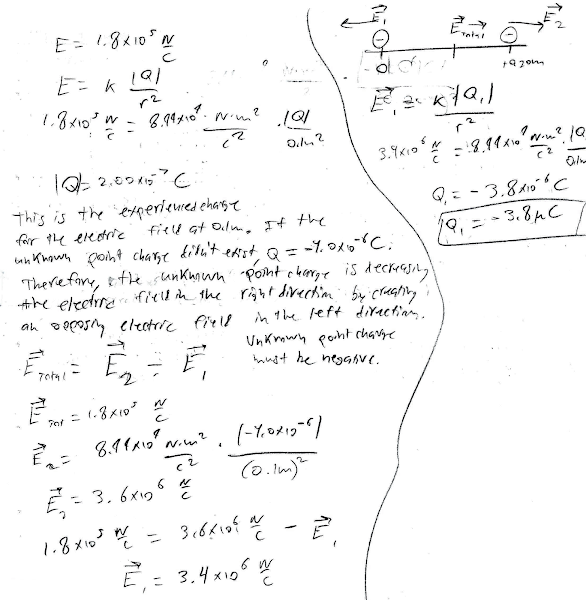A sample "v" response (from student 9224):## 20150611

### Physics final exam problem: switched-in voltmeter

Physics 205B Final Exam, spring semester 2015
Cuesta College, San Luis Obispo, CA

Cf. Giambattista/Richardson/Richardson, Physics, 2/e, Problems 18.31, 18.72, 18.73An ideal emf source is connected to two light bulbs (each with the same resistance R), a voltmeter, and an open switch. All of these components are ideal. Determine whether the voltmeter (magnitude) reading will increase, decrease, or remain constant when the switch is closed. Show your work and explain your reasoning using the properties of voltmeters, Kirchhoff's rules and Ohm's law.

• p:
Correct. Understands that:
1. when the switch is open, the voltmeter reading will be zero, as there is no potential difference between its terminals, and/or it is "not wired properly" and so will have a null/nonsense reading;
2. when the switch is closed, no current will flow through the voltmeter (as it has infinite resistance), but it is wired in parallel to the left light bulb, and will read the light bulb's voltage drop ΔV = IR,
thus closing the switch would result in a higher voltmeter reading.
• r:
Nearly correct, but includes minor math errors.
• t:
Nearly correct, but approach has conceptual errors, and/or major/compounded math errors.
• v:
Implementation of right ideas, but in an inconsistent, incomplete, or unorganized manner.
• x:
Implementation of ideas, but credit given for effort rather than merit. May confuse voltmeters with ammeters, and/or discusses how current will start to flow through voltmeter when switch is closed, etc.
• y:
Irrelevant discussion/effectively blank.
• z:
Blank.